Test: Introduction to Systems

# Test: Introduction to Systems

Test Description

## 10 Questions MCQ Test GATE Electrical Engineering (EE) 2023 Mock Test Series | Test: Introduction to Systems

Test: Introduction to Systems for Electronics and Communication Engineering (ECE) 2023 is part of GATE Electrical Engineering (EE) 2023 Mock Test Series preparation. The Test: Introduction to Systems questions and answers have been prepared according to the Electronics and Communication Engineering (ECE) exam syllabus.The Test: Introduction to Systems MCQs are made for Electronics and Communication Engineering (ECE) 2023 Exam. Find important definitions, questions, notes, meanings, examples, exercises, MCQs and online tests for Test: Introduction to Systems below.
Solutions of Test: Introduction to Systems questions in English are available as part of our GATE Electrical Engineering (EE) 2023 Mock Test Series for Electronics and Communication Engineering (ECE) & Test: Introduction to Systems solutions in Hindi for GATE Electrical Engineering (EE) 2023 Mock Test Series course. Download more important topics, notes, lectures and mock test series for Electronics and Communication Engineering (ECE) Exam by signing up for free. Attempt Test: Introduction to Systems | 10 questions in 30 minutes | Mock test for Electronics and Communication Engineering (ECE) preparation | Free important questions MCQ to study GATE Electrical Engineering (EE) 2023 Mock Test Series for Electronics and Communication Engineering (ECE) Exam | Download free PDF with solutions
 1 Crore+ students have signed up on EduRev. Have you?
Test: Introduction to Systems - Question 1

### A function f(t) is an even function, if for all values of (t) ( T is the time-period of the function)

Detailed Solution for Test: Introduction to Systems - Question 1

For even function, f(t) = f(-t)

For odd function, f(t) = -f(-t)

Test: Introduction to Systems - Question 2

### A control system transfer function is H(s) = 1/s3. Express its impulse response in terms of unit step signal

Detailed Solution for Test: Introduction to Systems - Question 2

Convolution in the time domain implies multiplication in S(or frequency) domain

The Laplace transform of any signal h(t) is given by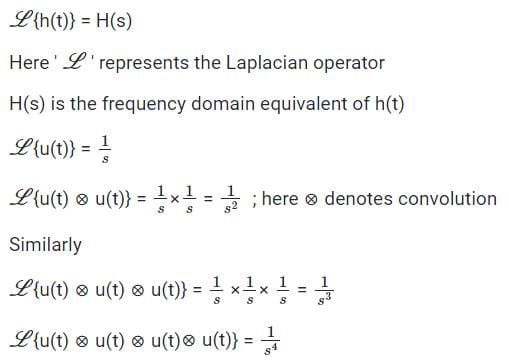So we observe that the H(s) = 1/s3, corresponds to a unit step signal convoluted with itself thrice.

Therefore the correct answer is option 1

Test: Introduction to Systems - Question 3

### The unit impulse response of a linear time invariant system is the unit step function u(t) for t > 0, the response of the system to an excitation e-at u(t), a > 0 will be

Detailed Solution for Test: Introduction to Systems - Question 3

Given: h(t) = u(t)
x(t) = e–at u(t)

∴ Y(s) = X(s) H(s) =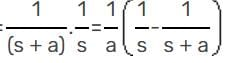y(t) = 1/a (1 – e– at) u(t)

Test: Introduction to Systems - Question 4

A system with an input x(t) and output y(t) is described by the relation: y(t) = tx(t). This system is

Detailed Solution for Test: Introduction to Systems - Question 4

y(t) = tx(t)

y1(t) = t.x1(t) = r1(t)

y2(t) = tx2(t) = r2(t)

y1(t) + y2(t) = t(x1(t) + x2(t))

= r1(t) + r2(t)    ∴ linear

y(t) = t.x(t)

y( t - to) = (t - to) x ( t - to)

and for delayed input signal,

y(t) = t x (t - to)

y(t) ≠ y( t-to)

∴ Time varying signal

Test: Introduction to Systems - Question 5

If a function f(t) u(t) is shifted to the right side by t0, then the function can be expressed as

Detailed Solution for Test: Introduction to Systems - Question 5

Since f(t) u(t) = f(t) for t > 0 also we know

u ( t - to) = 1, for t > to

Here in right side shifting that means to > 0

by property on shifting right side,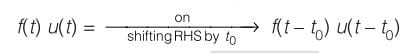Test: Introduction to Systems - Question 6

The impulse response of a causal, linear, time- invariant, continuous time system is h(t). The output y(t) of the same system to an input x(t). Where x(t) = 0 for t < -2 is

Detailed Solution for Test: Introduction to Systems - Question 6

Since, causal system

h(t) = 0 for t < 0

Input y(t) = x(t) * h(t)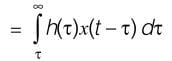h(τ) = 0 for τ < 0

x ( t - τ) = 0, for t - τ < - 2

∴ τ > f + 2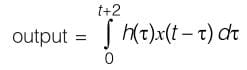Test: Introduction to Systems - Question 7

The unit step response of a system is given by (1 - eαt) u(t), the impulse response is given by

Detailed Solution for Test: Introduction to Systems - Question 7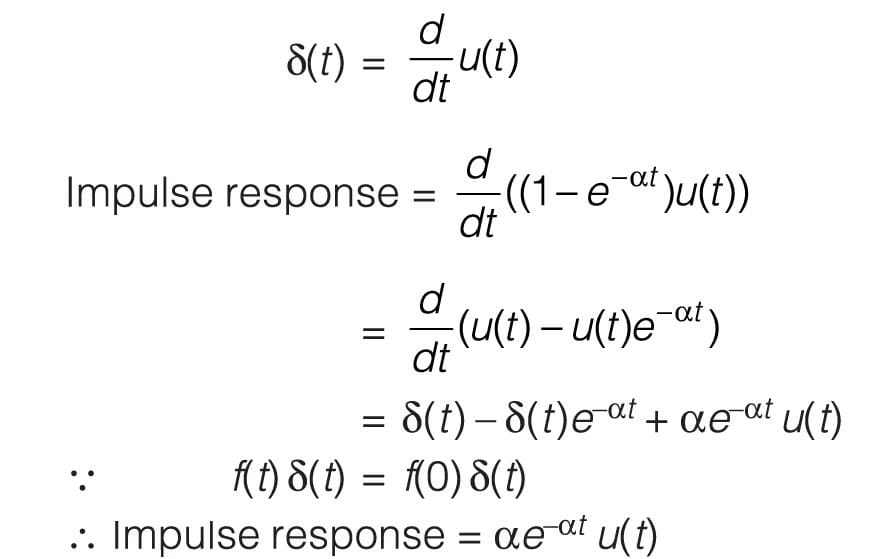Test: Introduction to Systems - Question 8

Figure I and Figure II, shows the input x(t) to a linear time invariant system and the impulse response h(t) of the system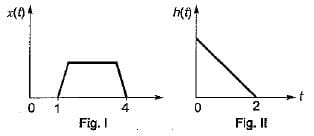the output of the system is zero everywhere except for the time interval.

Detailed Solution for Test: Introduction to Systems - Question 8

Given x(t) and h(t) y(t) = x(t) * h(t)

y(t) =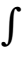x(τ) h(t – τ)dτ– ∞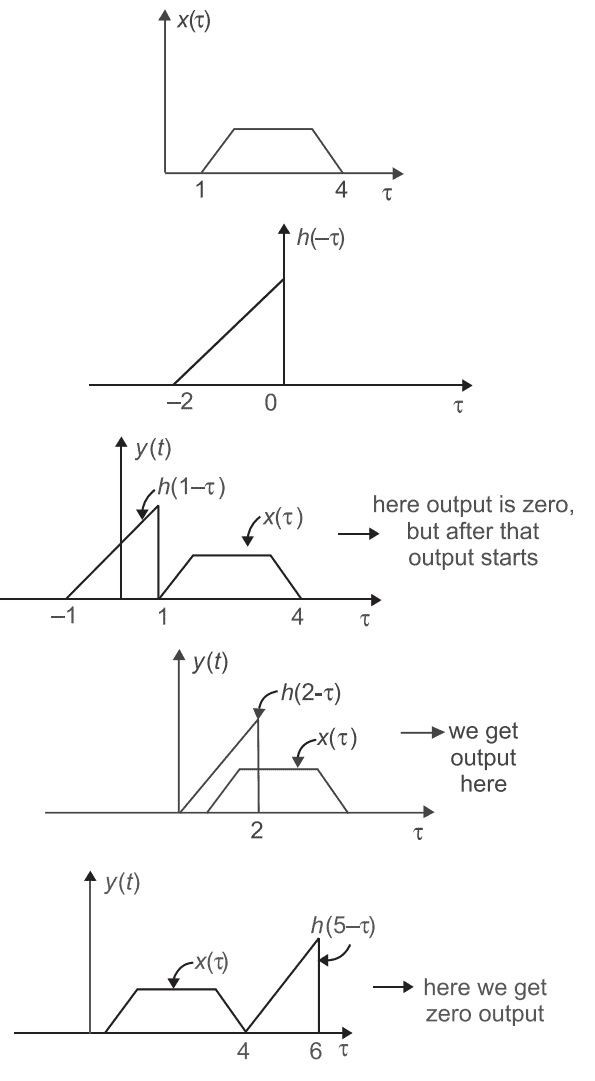Thus, we conclude that the output of the system is zero everywhere except for the time interval.
1 < t < 5

Test: Introduction to Systems - Question 9

A signal f(t) = cos8πt + 0.5cos4πt is instantaneously sampled. The maximum allowable value of sampling interval Ts in sec is

Test: Introduction to Systems - Question 10

The impulse response. h[n] of a linear time invariant system is given by h[n] = u[n + 3] + u[n - 3] - 2u[n - 7], the above system is

## GATE Electrical Engineering (EE) 2023 Mock Test Series

22 docs|274 tests
 Use Code STAYHOME200 and get INR 200 additional OFF Use Coupon Code
Information about Test: Introduction to Systems Page
In this test you can find the Exam questions for Test: Introduction to Systems solved & explained in the simplest way possible. Besides giving Questions and answers for Test: Introduction to Systems, EduRev gives you an ample number of Online tests for practice

## GATE Electrical Engineering (EE) 2023 Mock Test Series

22 docs|274 tests